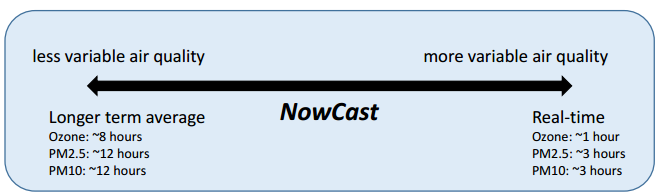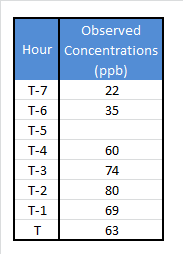# The NowCast for Ozone (obsolete as of 08/01/2019)

## Nowcast Overview

From the EPA’s AQI Technical Assistance Document page 7:

EPA uses the NowCast to approximate the complete daily AQI during any given hour. Even on days when the AQI forecast predicts unhealthy conditions, pollution levels may be lower and better for outdoor activities during some parts of the day. Providing current conditions gives people the power to take action to reduce outdoor activities and exposure when necessary and protect their health.

The NowCast calculation uses longer averages during periods of stable air quality and shorter averages when air quality is changing rapidly, such as during a fire. The NowCast allows current conditions maps to align more closely with what people are actually seeing or experiencing## Completeness Criteria for the NowCast and Clarification on Truncation vs. Rounding

• There is only 1 requirement for a valid NowCast calculation: 2 of the past 3 hourly data points must be valid.

• The Nowcast calculation uses the raw hourly concentrations (not truncated or rounded). The Nowcast values are then truncated to the nearest 1 ppb for ozone, 1 ug/m3 for PM10, and 0.1 ug/m3 for PM2.5. This truncated concentration is then converted to AQI using the AQI equation. The resulting AQI is rounded to the nearest whole number.

## Ozone NowCast Calculation

The ozone NowCast replaced the ozone surrogate (slope/intercept method) for real time reporting in early 2016. The ozone NowCast calculation is similar to the PM2.5 and PM10 NowCast calculations, with some slight differences (indicated by the bold text below). The process for calculating the ozone NowCast is as follows:

1. Compute the concentrations range (max-min) over the last 8 hours.

2. Divide the range by the maximum concentration in the 8 hour period to obtain the scaled rate of change.

3. Compute the weight factor by subtracting the scaled rate from 1.** There is no minimum weight factor** (PM2.5 and PM10 have a minimum weight factor of 0.5). There is a maximum weight factor of 1.

4. Multiply each hourly concentrations by the weight factor raised to the power of how many hours ago the concentration was measured (for the current hour, the factor is raised to the zero power).

5. Compute the NowCast by summing these products and dividing by the sum of the weight factors raised to the power of how many hours ago the concentration was measured.

Example Calculation1. Range: 80-22=58
2. Scaled rate of change: 58/80= .725
3. Weight Factor: 1-.725= .275
4. 63*(.275)0+69*(.275)1+80*(.275)2+74*(.275)3+60*(.275)4+Missing*(.275)5+35*(.275)6+22*(.275)7= 89.925
5. 89.925/ (.275)0+(.275)1+(.275)2+(.275)3+(.275)4+(.275)5+(.275)6+(.275)7=